﻿ A Look at Insertion Sort | kirupa.com

Color

Background

Done

# A Look at Insertion Sort

by kirupa   |   26 December 2014

A fun sorting algorithm is insertion sort. Have you ever played cards and needed to sort the cards in your hand?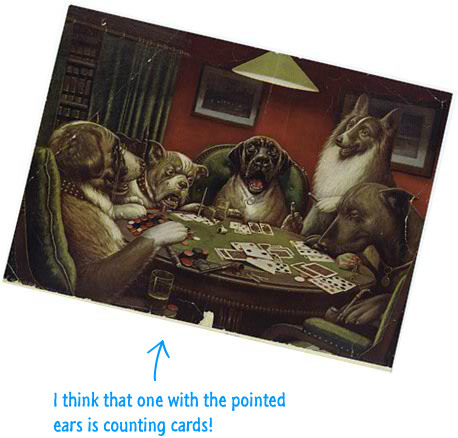The approach you (or your canine friend) use to sort a playing card from one part of your hand and move it to the other part is nearly identical to how insertion sort works. It is actually a tad bit more exciting than that sounds, so let's look at how insertion sort works in this article.

Onwards!

## How Insertion Sort Works

The way insertion sort works is pretty simple. Like all the great algorithms, it makes its way slowly from left to right on a collection of numbers you want to sort. As it is making its way through each number, it stops at each one (which I will call the active number) for some friendly conversation. This "friendly conversation" involves taking a look the active number and seeing if it is larger than any of the numbers to its left.

If insertion sort finds that the active number has larger numbers to its left, it takes the active number (probably over its shoulder) and keeps moving left until it finds the properly sorted place to insert it. All of this probably makes very little sense right now, so let's use some friendly bar shapes to help us out here.

Let's say we have some numbers that insertion sort is currently working on: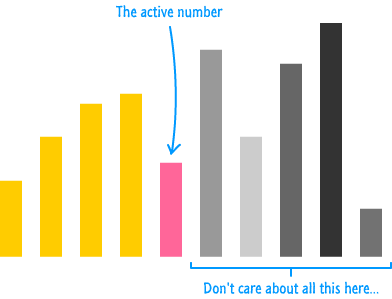The size of the bars indicate the magnitude of the number stored inside them, and our active number is the middle one that the arrow is pointing to. Everything to the left of the active number is already sorted from small to large. Everything to the right of the active number is irrelevant...for now.

Given what we've said is insertion sort's MO, it is going to look left to find a spot to insert our active number. It does so by dragging the active number to each number to its left and forcing it to ask "Am I bigger?":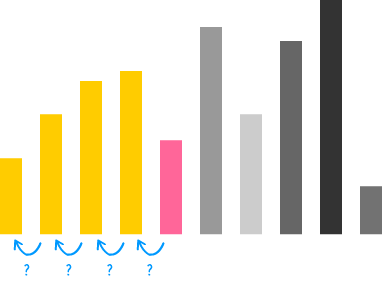When our algorithm gets to a point where the answer to that question is a "Yes", it means that our active number is at a spot where the number to its left is smaller:At this point, the active number plants itself at this location. Let's talk a little bit more about the details of what exactly just happened.

First, there is one word choice I need to call out. I deliberately omitted any reference to numbers to the right of the newly planted active number. The reason for that is subtle. If you have some numbers to the right that fall inside your partially sorted region, you kinda have some idea about them. If you look further right beyond your sorted region into unchartered territory, we have no idea what is going on there:The reason is pretty simple. We will never examine any of the bars beyond the partially sorted region until we make one of them the active number. At no point will we ever know what all of the remaining bars are. That's not a problem. The only thing we know is this: we have a partially sorted list right now, and within this partially sorted list, the bars are guaranteed to be sorted. Everything else is irrelevant.

As the algorithm makes its way right, more of the unchartered territory get revealed. With each shift to the right, we repeat this "Look Left" policy and insert each new active number into its proper place in the partially sorted region on the left. By the time you are at the end, all of the items will be properly sorted: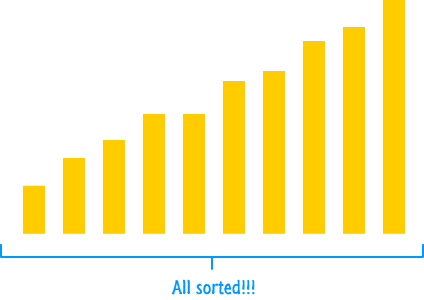Now that you've seen this, we'll look into how insertion sort works in greater detail and make fun of how ineffecient it is. Woohoo!

## Insertion Sort Walkthrough

In real life, you'll probably not be sorting bars. You'll be sorting numbers, so let's take what we've learned in the previous section and fully sort some numbers using insertion sort:These six numbers need some sorting, and we are going to use insertion sort to see how that will happen. To start with, we are going to skip the first number and focus on the second number. This active number (the number we are currently trying to sort) would be the number 3.Once we have your active number, we follow the "look left" approach that insertion sort operates under. This is the approach where it compares the active number against each number to the left of it until it hits a number it is larger than.

We only have one number to the left of us, so let's compare the 3 against the 5. Is the active number (3) greater than 5? The answer is no. So we move left. Since there is nothing more to the left, the active number gets inserted in its new home at the beginning: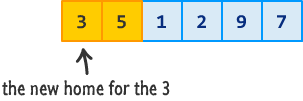Next, we move right and pick a new active number: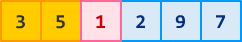That new active number would be the 1. Repeating the earlier process, let's look left. The 1 is not greater than 5. The 1 is not greater than 3. There is no other number to the left, so the 1 now gets inserted as the first item in the list: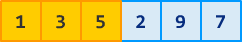Pretty simple, right? I'm going to speed this up a bit by skipping a lot of the explanations and just showing the results of our active number reaching its new destination for the remaining numbers: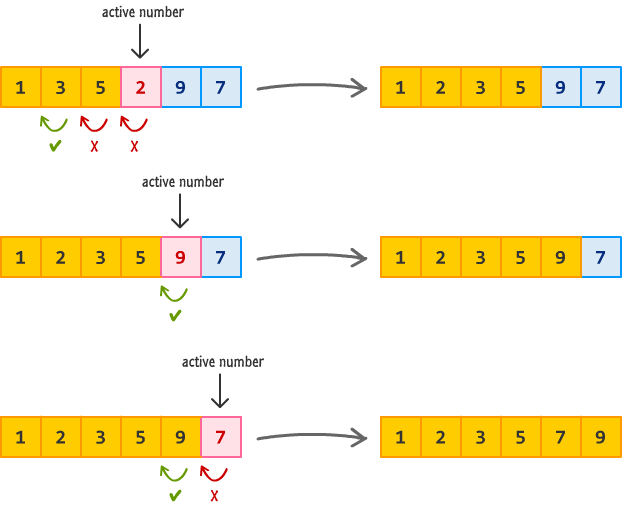That's all there is to it. The way insertion sort works is pretty simple once you get the basic approach it uses for taking the active number and inserting it in the correct location. We aren't done just yet. I now need to bore you by verbally describing the insertion sort algorithm. This is important so that you can tell your friends that you learned about insertion sort without just looking at pretty diagrams.

## Boring Algorithm Overview

Anyway, here is the insertion sort algorithm:

1. Start at the beginning and assume the first number is already sorted. The number we are going to focus on (aka the active number) is the second item.
2. With your active number in your grasp, see if it is less than or greater than the number that is before it:
1. If the active number is greater than the number to its left, do nothing. The ordering is correct… for now. Jump to Step 3.
2. If the active number is less than the number to its left, keep moving left. Keep moving over until your active number hits another number whose value is less than it. When that joyous moment happens, stay put and wedge the active number just next to that number.
3. It’s time to repeat the whole process with a new active number. Pick the next unsorted number and start all over from Step 2.

The performance characteristics of insertion sort are nothing to write home about. Let's look at why it is pretty slow.

At a bare minimum, it takes n operations to just go from one end of your data to the other: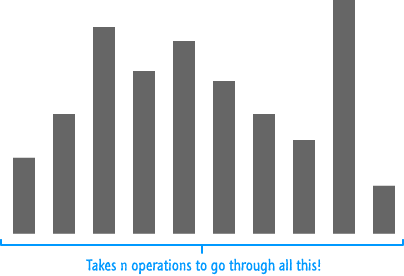Each operation to take an active number and insert it into the proper position on the left takes linear time: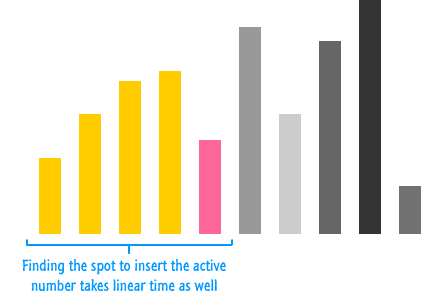You put all that together, you get an average running time of n2 where the linear time to through your numbers combined by the linear "look left" insertion at each point make it a pretty slow algorithm. Now, it isn't all bad news for all you insertion sort afficionados, though! It isn't memory intensive at all. It takes up a constant amount of memory, so keep insertion sort at the top of your pile if you need to sort numbers (slowly) but are memory constrained.

## The JavaScript Implementation

The last thing before we say our tearful goodbyes is taking a look at a working JavaScript implementation of insertion sort:

```function insertionSort(input) {
var activeNumber;

for (var i = 1; i < input.length; i++) {
activeNumber = input[i];

for (var j = i - 1; j >= 0; j--) {

if (input[j] > activeNumber) {
input[j + 1] = input[j];
} else {
break;
}
}
input[j + 1] = activeNumber
}
}

var myinput = [24, 10, 17, 9, 5, 9, 1, 23, 300];
insertionSort(myinput);

This implementation is a near direct translation of the English explanations and diagrams you saw earlier. The main thing to note is that there is the outer loop that is responsible for travelling through your data. The inner loop is responsible for taking each active number, looking left at the already sorted data, and specifying the spot that that the active number will be inserted into.

## Conclusion

Insertion sort is not very efficient from a speed point of view. It is, however, very efficient from a memory point of view. To see how insertion sort compares with other sort algorithms, check out the following table:

Name Best Average Worst Memory
Quicksort n log n n log n n2 log n (average)
Mergesort n log n n log n n log n n (worst case)
Heapsort n log n n log n n log n 1
Tinsort n n log n n log n n
Bubble sort n n2 n2 1
Selection sort n2 n2 n2 1
Insertion sort n n2 n2 1

You can find a more detailed comparison of these sorting algorithms as well as many others over on the Wikipedia section fully dedicated to this. Overall, stay clear of insertion sort unless you are sorting a small amount of numbers or really need to take advantage of its sweet constant memory usage.

Just a final word before we wrap up. If you have a question and/or want to be part of a friendly, collaborative community of over 220k other developers like yourself, post on the forums for a quick response!